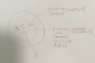# Calculate the electric field in all of space for a non conducting sphere

I apologize for any confusion,In summary, Gauss's Law can be used to find the electric field in all space for a non-conducting sphere with volumetric charge distribution ρ=ρ0r3 and radius, R.

## Homework Statement

A) Use Gauss's Law to derive the electric field in all space for a non-conducting sphere with volumetric charge distribution ρ=ρ0r3 and radius, R.
B) Repeat when there is a concentric spherical cavity within the non conducting sphere with radius, A.

## Homework Equations

∫E⋅dA=Qenclosed0
ρ=Q/V (charge density)

## The Attempt at a Solution

Im struggling with part B more than A, but since B uses part A's answer (in a way) I will show both my attempts

A) I drew a picture (sphere.jpg attached) and will use words to explain what I am doing.
My Gaussian Surface has radius, A.
I will be finding dQ of a small spherical piece of the sphere, the radius of this small spherical piece is, r, and has thickness, dr.

First find dQ
we know that ρ=Q/V →dQ=ρdV since ρ=ρ0r3 and dV=(4πr2)(dr) let's plug those into our dQ:
dQ=(ρ0r3)(4πr2)(dr)

We can now find our Qenclosed of gaussian surface.
My below integral's limits go from 0→a (a being the radius of my gaussian surface)
Qenclosed=∫dQ=∫ (ρ0r3)(4πr2)(dr)=4πρ0∫r4→once evaluated= (4πρ0a5)/5 (this is our Qenclosed)
Now we can use gauss's law to find electric field
E(4πa2)=(4πρ0a5)/(5ε0)
and finally:
E=(ρa3)/(5ε0)→E(r)=ρr3)/(5ε0)r(hat)
This is the answer if you are inside the sphere at some distance r<R

Now for outside the sphere:

We can go to the Qenclosed and just change the limits, instead of integrating from 0→a, we can integrate from 0→R. If we do this our Qenclosed becomes:

Qenclosed=(4πρ0R5)/5

My new gaussian surface has radius a'

E(4πa'2)=(4πρ0R5)/(5ε0)
solving for E:
E=(ρ0R5)/(5a'2ε0)

Now for part B

Again, new limits for Qenclosed, the limits now are going from A→a
our new Qenclosed=[(4πρ0a5)/(5)]-[(4πρ0A5)/(5)]
and our new E=[(ρ0a3)/(5ε0)]-[(ρ0A5)/(5a2ε0)

and outside our Qenclosed=[(4πρ0R5)/(5)]-[(4πρ0A5)/(5)]
and our new E=[(ρ0R5)/(5a2ε0)]-[(ρ0A5)/(5a2ε0)

Hope that was clear enough, please ask for more steps if I am wrong.
thanks!

#### Attachments

•Sphere.jpg
17.9 KB · Views: 238
Hello,
Qenclosed=∫dQ=∫ (ρ0r3)(4πr2)(dr)=4πρ0∫r4→once evaluated= (4πρ0a5)/5
How do you do this ?

••